## Abstract

In the present paper, an analysis has been performed to study the influence of induced magnetic field on the transient free convective flow of an electrically conducting and viscous incompressible fluid over a vertical cone. The coupled nonlinear partial differential equations governing the transient flow have been solved numerically by using the implicit finite difference method of Crank–Nicolson type. The influence of magnetic parameter, magnetic Prandtl number and semi-vertical angle of the cone on the velocity and induced magnetic field profiles has been illustrated graphically. Also, the local as well as average skin-friction and Nusselt number has been presented graphically. For result validation, we have done a comparative study and the present results are found to be in very good agreement with available results.

## Keywords

Induced magnetic field; Magnetic parameter; Magnetic Prandtl number; Vertical cone

## Nomenclature

${\textstyle C_{p}}$- specific heat at constant pressure

g- acceleration due to gravity

${\textstyle H_{0}}$- constant applied magnetic field

${\textstyle H_{1}^{'}}$- induced magnetic field component along surface of cone

${\textstyle H_{2}^{'}}$- induced magnetic field component perpendicular to surface of cone

${\textstyle H_{1}}$- dimensionless induced magnetic field component along surface of cone

${\textstyle H_{2}}$- dimensionless induced magnetic field component perpendicular to surface of cone

L- characteristic length

M- magnetic parameter

Nu- Nusselt number

${\textstyle {Nu}_{av}}$- average Nusselt number

Pm- magnetic Prandtl number

Pr- Prandtl number

${\textstyle r^{'}}$- local radius of the cone

${\textstyle T^{'}}$- temperature of the fluid

T- dimensionless temperature

${\textstyle t^{'}}$- time

t- dimensionless time

${\textstyle u^{'}}$- velocity component along surface of cone

${\textstyle v^{'}}$- velocity component perpendicular to surface of cone

u- dimensionless velocity component along surface of cone

v- dimensionless velocity component perpendicular to surface of cone

${\textstyle x^{'}}$- distance along surface of cone

${\textstyle y^{'}}$- distance perpendicular to surface of cone

x- dimensionless distance along surface of cone

y- dimensionless distance perpendicular to surface of cone

### Greek symbols

${\textstyle \beta }$- coefficient of thermal expansion

${\textstyle {\mu }_{0}}$- magnetic permeability

${\textstyle \nu }$- kinematic viscosity

${\textstyle \eta }$- magnetic diffusivity

${\textstyle \phi }$- semi vertical angle of cone

${\textstyle \rho }$- density

${\textstyle \tau }$- local skin-friction

${\textstyle {\tau }_{av}}$- average skin-friction

${\textstyle \kappa }$- thermal conductivity of fluid

## 1. Introduction

Free convective flow of an electrically conducting fluid in the presence of a magnetic field has been investigated substantially by many researchers because of its wide appearance in many industrial as well as technological applications. Heat transfer over a vertical cone in convective process is used for designing of engineering and scientific equipments such as nuclear waste disposal, nuclear reactor cooling systems, geothermal reservoirs . In the present article, the system we are studying, a vertical cone with apex at the origin. The applied magnetic field is perpendicular to the surface along y direction crossing the fluid motion. The resultant of this, the induced magnetic field is generated as shown in figure. Similarity solutions for axi-symmetrical problems for steady natural convective laminar flow over a vertical cone have been developed by many researchers , , , ,  and . Pop and Takhar  have analyzed the compressibility effects of the laminar free convective flow from a vertical cone while Watanabe  and Hossain and Paul  have studied the effect of different types of boundary conditions over a cone. Since, unsteady mixed convective flows do not specifically produce similarity solution; therefore, several flow problems have been analyzed, in which the non-similarity solutions were considered. There are a number of factors due to which unsteadiness and non-similarity occur in fluid flows such as velocities at edge of boundary layer, body curvature.

Anilkumar and Roy  have considered the unsteady mixed convection flow on a rotating cone in a rotating fluid due to the combined effects of thermal and mass diffusion. They obtained numerical results for the skin friction coefficients, Nusselt number and Sherwood number and presented the influence of various parameters on the velocity, temperature and concentration profiles. They also added that the self-similar solution can only exist, if the angular velocities at the edge and at the cone wall inversely vary as a linear function of time. Al-Harbi  performed the numerical analysis of natural convection heat transfer with effect of variable viscosity and thermal radiation from a cone and wedge in porous media and showed the effects of different pertinent parameters on the velocity, temperature and rate of heat transfer. Bapuji et al.  considered the finite difference analysis of laminar free convection flow past a non-isothermal vertical cone. They came to the conclusion that the difference between temporal maximum values and steady state values for both velocity and temperature becomes less when Prandtl number increases and the impact of Prandtl number on the local skin-friction and the local Nusselt number increases along the surface from the apex.

In all these studies, magnetic field has not been considered. It is found that magnetic field influences heat transfer processes of electrically conducting fluid flows. It has applications in industrial and technological fields such as metal coating, crystal growth, reactor cooling. Therefore, many researchers have shown their interest in studies of MHD free convective flow. MHD flow with heat flux is widely used in geothermal, geophysical and engineering fields. The geothermal gasses being electrically conducting are affected by magnetic field Bapuji and Chamkha  and Prakash et al. . In many situations, variations in temperature become too large that variation in viscosity in thermal boundary layers cannot be neglected. This problem commonly appears in hot rolling process, production of glass fiber, plastic films drawing, drawing of wire and gluing of labels on hot bodies Kumar and Sivaraj . In practical metallurgical processing where rates of extinguishing employ a significant effect on the finishing structure of products and unsteady hydromagnetic flows plays a big role. Takhar et al.  scrutinized the unsteady mixed convection flow from a rotating vertical cone with magnetic field. They concluded that tangential and azimuthal skin-friction coefficients and Nusselt number increase with time when angular velocity of cone increases.

Unsteady laminar heat and mass transfer from a rotating vertical cone with a magnetic field and heat generation or absorption effects were numerically investigated by Chamkha and Al-Mudhaf . They found that the increase in buoyancy force increases the skin friction coefficients and the Nusselt number. Ece  studied free convection flow about a cone under mixed thermal boundary conditions and a magnetic field and he showed that the magnetic field retards the velocity profiles and expands the temperature profiles by increasing both the surface shear stress and temperature. The effect of different pertinent parameters on the velocity, temperature and rate of heat transfer was also discussed. Further, Ece  analyzed free convection flow about a vertical spinning cone under a magnetic field. He observed that the spin of the body expands the velocity profiles by increasing the skin friction and squeezes the temperature profiles toward the surface by increasing the surface heat flux for the specified surface temperature case and decreasing surface temperature for the specified heat flux case. The effect of mixed thermal boundary conditions and magnetic field on free convection flow about a cone in micropolar fluids was examined by Modather et al.  and they obtained transformation related to the similarity solutions of the boundary layer velocity, microrotation, and temperature profiles. Mahdy et al.  deliberated the study of the influence of magnetic field on natural convective flow near a wavy cone in porous media. It was found that magnetic field slows down the heat transfer process by decreasing the local Nusselt number and increasing the fluid temperature. With the combined effect of heat transfer many interesting flow problems have been studied in magnetohydrodynamic convection flows. Vajravelu et al.  have investigated axisymmetric magnetohydrodynamic flow and heat transfer at a non-isothermal stretching cylinder and found that the transverse curvature increases the horizontal velocity and the temperature fields while increase in magnetic parameter decreases the velocity boundary layer thickness. Natural convective flow and heat transfer enhancement of a nanofluid past a truncated cone with magnetic field effect were carried out by Ahmed and Mahdy  and they found that spherical nanoparticle enhance the heat transfer rate most strongly. Kumar and Singh  have investigated transient MHD natural convection past a vertical cone having ramped temperature on the curved surface of the cone. They observed that effect of magnetic field and semi-vertical angle of the cone on the velocity profiles is found to decrease it.

These all studies were restricted to the case in which induced magnetic field has been neglected. Induced magnetic field strongly influences the flow formation and heat transfer. To control the flow formation rate more accurately, it is necessary to take induced magnetic field into consideration. The induced magnetic field has many important applications in the experimental and theoretical studies of MHD flow due to its use in many industrial and technological phenomena , , , , , ,  and . Therefore, as an advanced step in progress of magnetohydromagnetic flow it is significant to investigate effect of induced magnetic field on unsteady MHD free convective flow past a body  and . As a step toward the subsequent development of studies of magnetohydromagnetic flows it is interesting as well as useful to investigate the effect of induced magnetic field on transient free convective flow through a cone. In the present study, we are interested to find the numerical solution of the transient free convective flow through a vertical cone under the influence of induced magnetic field. The governing equations are solved numerically by using the Crank–Nicolson method and the effects of various significant parameters on the flow, heat transfer and induced magnetic field characteristics are presented in both graphical and tabular forms.

## 2. Problem formulation

We consider the transient hydromagnetic free convective flow of an electrically conducting and viscous incompressible fluid through axi-symmetric cone in two-dimensional system. The orthogonal system (${\textstyle x^{'}{\mbox{,}}y^{'}{\mbox{,}}z^{'}}$) is considered, where ${\textstyle x^{'}}$ – is measured along the lateral side of the cone, ${\textstyle y^{'}}$ – axis perpendicular to the circular section and ${\textstyle z^{'}}$ – axis normal to the cone surface with apex at the origin. Let ${\textstyle u^{'}}$ and ${\textstyle v^{'}}$ be the velocity components along ${\textstyle x^{'}}$ (tangential) and ${\textstyle y^{'}}$ (circumferential) direction as illustrated in Fig. 1. Here, ${\textstyle r^{'}}$ is the radial distance from the surface element to the axis of symmetry and ${\textstyle \phi }$ is the semi-vertical angle of the cone. A uniform applied magnetic field of strength ${\textstyle H_{0}}$ is acting parallel to the ${\textstyle y^{'}}$-axis. Resultant of this, the induced current is generated in the fluid. The induced current modifies the uniform applied magnetic field as (${\textstyle H_{1}^{'}{\mbox{,}}H_{2}^{'}{\mbox{,}}0}$). Initially, ${\textstyle \left(t^{'}\leqslant 0\right)}$, both the axi-symmetric cone and an ambient fluid are assumed to be at undisturbed temperature ${\textstyle T_{\infty }^{'}}$, when ${\textstyle t^{'}>0}$, the surface temperature of the cone will be raised as a constant temperature ${\textstyle T_{w}^{'}}$, greater than the ambient temperature ${\textstyle T_{\infty }^{'}}$.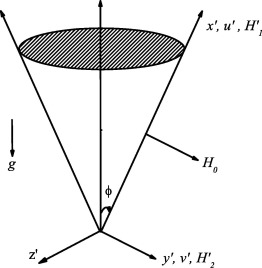Figure 1. Geometry of the problem.

According to the physical model under consideration of Boussinesq approximation, the governing two-dimensional boundary layer equations are derived as follows , ,  and :

 ${\frac {\partial (r^{'}u^{'})}{\partial x^{'}}}+$${\frac {\partial (r^{'}v^{'})}{\partial y^{'}}}=$$0{\mbox{,}}$
(1)

 ${\frac {\partial (r^{'}H_{1}^{'})}{\partial x^{'}}}+$${\frac {\partial (r^{'}H_{2}^{'})}{\partial y^{'}}}=$$0{\mbox{,}}$
(2)

 ${\frac {\partial u^{'}}{\partial t^{'}}}+u^{'}{\frac {\partial u^{'}}{\partial x^{'}}}+$$v^{'}{\frac {\partial u^{'}}{\partial y^{'}}}=\nu {\frac {{\partial }^{2}u^{'}}{\partial y^{{'}2}}}+$$g\beta (T^{'}-T_{\infty }^{'})Cos\quad \phi +{\frac {{\mu }_{0}}{4\pi \rho }}\left(H_{1}^{'}{\frac {\partial H_{1}^{'}}{\partial x^{'}}}+\right.$$\left.H_{2}^{'}{\frac {\partial H_{1}^{'}}{\partial y^{'}}}\right){\mbox{,}}$
(3)

 ${\frac {\partial H_{1}^{'}}{\partial t^{'}}}+u^{'}{\frac {\partial H_{1}^{'}}{\partial x^{'}}}+$$v^{'}{\frac {\partial H_{1}^{'}}{\partial y^{'}}}=$$\eta {\frac {{\partial }^{2}H_{1}^{'}}{\partial y^{{'}2}}}+$$\left(H_{1}^{'}{\frac {\partial u^{'}}{\partial x^{'}}}+\right.$$\left.H_{2}^{'}{\frac {\partial u^{'}}{\partial y^{'}}}\right){\mbox{,}}$
(4)

 ${\frac {\partial T^{'}}{\partial t^{'}}}+u^{'}{\frac {\partial T^{'}}{\partial x^{'}}}+$$v^{'}{\frac {\partial T^{'}}{\partial y^{'}}}={\frac {\kappa }{\rho C_{p}}}{\frac {{\partial }^{2}T^{'}}{\partial y^{{'}2}}}$
(5)

The initial and boundary conditions for the considered problem are as follows:

 $t^{'}\leqslant 0:\quad u^{'}=0{\mbox{,}}\quad v^{'}=$$0{\mbox{,}}\quad T^{'}=T_{\infty }^{'}{\mbox{,}}\quad H_{1}^{'}=$$0{\mbox{,}}\quad H_{2}^{'}=0\quad for\quad 0\leqslant x^{'}{\mbox{,}}y^{'}<\infty {\mbox{;}}$

 $t^{'}>0:\quad {\begin{array}{llllll}u^{'}=0{\mbox{,}}&T^{'}=T_{\infty }^{'}{\mbox{,}}&H_{1}^{'}=0&&&at\quad x^{'}=0{\mbox{;}}\\u^{'}=0{\mbox{,}}&v^{'}=0{\mbox{,}}&T^{'}=T_{w}^{'}{\mbox{,}}&H_{1}^{'}=H_{0}&H_{2}^{'}=0&at\quad y^{'}=0{\mbox{;}}\\u^{'}\rightarrow 0{\mbox{,}}&T^{'}\rightarrow T_{\infty }^{'}{\mbox{,}}&H_{1}^{'}\rightarrow H_{0}&&&as\quad y^{'}\rightarrow \infty \end{array}}$
(6)

The non-dimensional variables are defined as follows:

 $t={\frac {\nu t^{'}}{L^{2}}}{\mbox{,}}\quad (x{\mbox{,}}y)=$${\frac {\left(x^{'}{\mbox{,}}y^{'}\right)}{L}}{\mbox{,}}\quad (u{\mbox{,}}v)=$${\frac {(u^{'}{\mbox{,}}v^{'})L}{\nu }}\quad T={\frac {T^{'}-T_{\infty }^{'}}{T_{w}^{'}-T_{\infty }^{'}}}{\mbox{,}}\quad (H_{1}{\mbox{,}}H_{2})=$${\frac {\left(H_{1}^{'}{\mbox{,}}H_{2}^{'}\right)}{H_{0}}}$
(7)

Eqs. (1), (2), (3), (4) and (5) in non-dimensional form can be expressed by using Eq. (7) as follows:

 ${\frac {\partial u}{\partial x}}+{\frac {\partial v}{\partial y}}+$${\frac {u}{x}}=0{\mbox{,}}$
(8)

 ${\frac {\partial H_{1}}{\partial x}}+{\frac {\partial H_{2}}{\partial y}}+$${\frac {H_{1}}{x}}=0{\mbox{,}}$
(9)

 ${\frac {\partial u}{\partial t}}+u{\frac {\partial u}{\partial x}}+$$v{\frac {\partial u}{\partial y}}={\frac {{\partial }^{2}u}{\partial y^{2}}}+$$T\quad Cos\quad \phi +M\left(H_{1}{\frac {\partial H_{1}}{\partial x}}+\right.$$\left.H_{2}{\frac {\partial H_{1}}{\partial y}}\right)$
(10)

 ${\frac {\partial H_{1}}{\partial t}}+u{\frac {\partial H_{1}}{\partial x}}+$$v{\frac {\partial H_{1}}{\partial y}}={\frac {1}{Pm}}{\frac {{\partial }^{2}H_{1}}{\partial y^{2}}}+$$\left(H_{1}{\frac {\partial u}{\partial x}}+H_{2}{\frac {\partial u}{\partial y}}\right)$
(11)

 ${\frac {\partial T}{\partial t}}+u{\frac {\partial T}{\partial x}}+$$v{\frac {\partial T}{\partial y}}={\frac {1}{Pr}}{\frac {{\partial }^{2}T}{\partial y^{2}}}{\mbox{.}}$
(12)

The initial and boundary conditions (6) in non-dimensional form are obtained as follows:

 $t\leqslant 0:\quad u=0{\mbox{,}}\quad v=0{\mbox{,}}\quad T=$$0{\mbox{,}}\quad H_{1}=0{\mbox{,}}\quad H_{2}=0\quad at\quad 0\leqslant x{\mbox{,}}y<\infty {\mbox{;}}\quad \quad$

 $t>0:\quad {\begin{array}{llllll}u=0{\mbox{,}}&&T=0{\mbox{,}}&H_{1}=0{\mbox{,}}&&at\quad x=0{\mbox{;}}\\u=0{\mbox{,}}&v=0{\mbox{,}}&T=1{\mbox{,}}&H_{1}=1{\mbox{,}}&H_{2}=0{\mbox{,}}&at\quad y=0{\mbox{;}}\\u\rightarrow 0{\mbox{,}}&&T\rightarrow 0{\mbox{,}}&H_{1}\rightarrow 0&&as\quad y\rightarrow \infty \end{array}}$
(13)

In the non-dimensional process, we get the non-dimensional parameters Prandtl number ${\textstyle \left(Pr\right)}$, magnetic Prandtl number ${\textstyle \left(Pm\right)}$, magnetic parameter ${\textstyle \left(M\right)}$ and the characteristic length L which are defined as

 $Pr={\frac {\nu \rho C_{p}}{\kappa }}{\mbox{,}}\quad Pm={\frac {\eta }{\nu }}{\mbox{,}}\quad M=$${\frac {{H_{0}}^{2}L^{2}{\mu }_{0}}{{\nu }^{2}4\pi \rho }}{\mbox{,}}\quad L=$${\left[{\frac {{\nu }^{2}}{\left\{g\beta (T_{w}^{'}-T_{\infty }^{'})\right\}}}\right]}^{1/3}{\mbox{.}}$
(14)

and ${\textstyle r^{'}=x^{'}Sin\quad \phi }$, where the other parameters are defined in nomenclature.

## 3. Numerical solution procedure

The transport Eqs. (8), (9), (10), (11) and (12) are highly nonlinear in nature and their solutions subject to the initial and boundary condition (13) have been obtained numerically. To facilitate this, the partial differential equations are first transformed into finite difference equations by employing a uniform grid discretization. The solutions of the transformed equations have been solved by implicit finite difference method of Crank–Nicolson type. Finally, the system of linear algebraic equations, expressed tridiagonally has been solved by Thomas algorithm. In the computational procedure, the physical domain is converted into computational domain as a rectangle frame of lines indicating ${\textstyle x_{min}=0{\mbox{,}}\quad x_{max}=1{\mbox{,}}\quad y_{min}=}$$0$ and ${\textstyle y_{max}=20}$ (as shown in Fig. 2) where ${\textstyle y_{max}}$ corresponds to ${\textstyle y\rightarrow \infty }$ which lies very far from the boundary layers.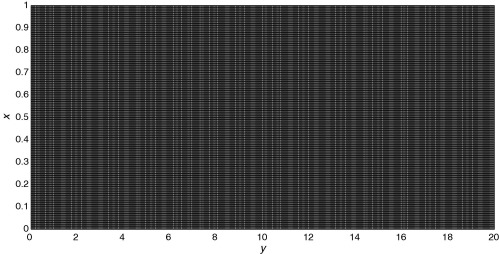Figure 2. The generated grid system.

To obtain efficient and tolerable results in the simulation process, a grid independent test has been performed for the steady state values of the velocity, temperature and induced magnetic field which is shown in Fig. 3. The values obtained in the iteration process with grid system of ${\textstyle 20\times 200}$ differ in the third decimal place from the grid systems of ${\textstyle 30\times 300}$ and ${\textstyle 40\times 400}$. Hence, for obtaining better results, we have considered ${\textstyle 21\times 201}$ grid points in the numerical computation with the fixed values of ${\textstyle Pr=0.71}$ (for air). The mesh sizes in the x and y   direction are taken as ${\textstyle \Delta x=0.05}$ and ${\textstyle \Delta y=0.05}$ respectively as shown in Fig. 2 with time step ${\textstyle \Delta t=0.01}$.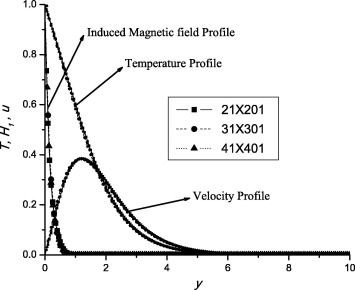Figure 3. Grid independent test for temperature, induced magnetic field and velocity profiles with ${\textstyle Pr=0.71}$ at steady state.

During any one time step, the computed values of the previous time step have been used for the coefficients ${\textstyle u{\mbox{,}}v{\mbox{,}}H_{1}{\mbox{,}}H_{2}}$ and T appearing in Eqs. (8), (9), (10), (11) and (12). At the end of each time step, first we have computed the temperature field and then the evaluated values are employed to obtain the velocity components in x and y directions respectively and finally the induced magnetic field components are obtained. The unsteady values of the components of velocity, induced magnetic field and temperature field for a desired time have been obtained by taking required number of iterations. The steady state numerical solutions have been obtained for the velocity, induced magnetic and temperature fields when the following convergence criterion is satisfied

 $\sum _{i{\mbox{,}}j}\left|{\frac {{\Phi }_{i{\mbox{,}}j}^{n+1}-{\Phi }_{i{\mbox{,}}j}^{n}}{{\Phi }_{i{\mbox{,}}j}^{n+1}}}\right|<{10}^{-5}{\mbox{,}}$
(15)

where ${\textstyle {\Phi }_{i{\mbox{,}}j}^{n}}$ stands for the temperature, velocity or induced magnetic field. The superscripts denote the values of the dependent variables after the n  th and ${\textstyle \left(n+1\right)}$th iterations of time ${\textstyle t(=n\Delta t)}$ respectively, whereas the subscripts i and j indicate grid location in xy   plane, where ${\textstyle x=i\Delta x}$ and ${\textstyle y=j\Delta y}$ with ${\textstyle \Delta x{\mbox{,}}\Delta y}$ and ${\textstyle \Delta t}$ the mesh size in ${\textstyle x{\mbox{,}}y}$ and t directions respectively. The generated grid system is shown in Fig. 2. In order to validate the accuracy of our simulation procedure, we have compared our results by neglecting the effect of magnetic field with the corresponding results in Hering  and Bapuji et al.  and found to be in good agreement.

Other important result of practical importance is the skin-friction. By using the computed values of the velocity field, the local skin-friction (${\textstyle \tau }$) and the average skin-friction (${\textstyle {\tau }_{av}}$) in non-dimensional form on the curved surface of the cone are obtained as follows:

 ${\tau ={\frac {\partial u}{\partial y}}}_{y=0}{\mbox{,}}$
(16)
 ${\tau }_{av}={\frac {1}{x_{max}}}{\int }_{0}^{x_{max}}\tau \quad dx{\mbox{.}}$
(17)

In engineering applications, one of the important characteristics of the flow is the rate of heat transfer over the cone surface. This is estimated by the values of the Nusselt number (Nu).

 ${Nu=-{\frac {\partial T}{\partial y}}}_{y=0}{\mbox{.}}$
(18)

Also, the overall heat transfer rate expressed by average Nusselt number (${\textstyle {Nu}_{av}}$) at the surface of the cone, is obtained as

 ${Nu}_{av}={\frac {1}{x_{max}}}{\int }_{0}^{x_{max}}Nu\quad dx{\mbox{.}}$
(19)

## 4. Results and discussions

With a view to see into the physical insight of the problem, numerical computations are carried out for the obtained physical parameters, magnetic Prandtl number (Pm), magnetic parameter (M  ), semi-vertical angle (${\textstyle \phi }$) of the cone and time parameter (t) on the velocity, induced magnetic field, skin-friction and Nusselt number profiles. In the entire computational procedure, we have assigned the common values to these parameters as Pm = 50, M = 0.5 and ${\textstyle \phi }$ = 15. The effects are shown with the help of graphs Figure 4, Figure 5, Figure 6, Figure 7, Figure 8, Figure 9, Figure 10, Figure 11, Figure 12, Figure 13, Figure 14 and Figure 15 on the various fields of considered model as well as a comparative study to achieve the steady state time, as shown in Table 2.

For result verification, we have compared the values of local skin-friction and local Nusselt number obtained in the present case with those of Bapuji et al.  and Hering  with the help of Table 1. From Table 1, it can be seen that the computed values for the local Nusselt number and local skin-friction are in excellent agreement for the case, in which induced magnetic field is negligible at a fixed cross section x = 1.

Table 1. Comparison of local skin-friction and local Nusselt number values at ${\textstyle x=1}$ with Bapuji et al.  and Hering  at steady-state.
Local skin-friction Local Nusselt number
Bapuji et al.  Hering  Present paper Bapuji et al.  Hering  Present paper
Pr ${\textstyle {\tau }_{x}/{Gr}_{L}^{3/4}}$ ${\textstyle f^{''}(0){\sqrt {Pr}}}$ ${\textstyle \tau }$ ${\textstyle {Nu}_{x}/{Gr}^{1/4}}$ ${\textstyle {\theta }^{'}(0){\sqrt {Pr}}}$ Nu
0.03 1.2368 1.2444 1.2103 0.1244 0.1244 0.1396
0.10 1.0911 1.096 1.0891 0.2115 0.2113 0.2139
0.70 0.8168 0.8195 0.8152 0.4529 0.5392 0.4565
1.0 0.7668 0.7694 0.7647 0.5129 0.5104 0.5170

Fig. 4 illustrates the variation of tangential velocity distribution of the fluid with respect to y   at a fixed cross section ${\textstyle x=1.0}$ for different values of the magnetic Prandtl number (Fig. 4(a)), magnetic parameter (Fig. 4(b)) and semi-vertical angle (Fig. 4(c)) respectively. Fig. 4(a) reveals that magnetic Prandtl number decreases the boundary layer thickness. As magnetic Prandtl number increases, viscosity of fluid increases and fluid becomes more thicker and consequently the boundary layer thickness decreases. It can also be observed that at the peak values of velocity, the influence of Pm becomes negligible. Also, the peak values of velocity shift away from the surface of the cone with increase in time. From Fig. 4(b), we can notice that as the value of magnetic parameter is increased, the momentum boundary layer thickness increases. Also, the velocity profiles near the cone surface become diminished. The maximum value of the velocity profiles is attained for higher values of M. Here also, the peak values shift away from the cone surface with increase in time. Fig. 4(c) shows influence of semi-vertical angle on tangential velocity. Fig. 4(c) depicts that as the values of ${\textstyle \phi }$ are increased the velocity profiles get reduced and boundary layer becomes expanded. Here, the velocity profiles attain maximum values for low values of ${\textstyle \phi }$.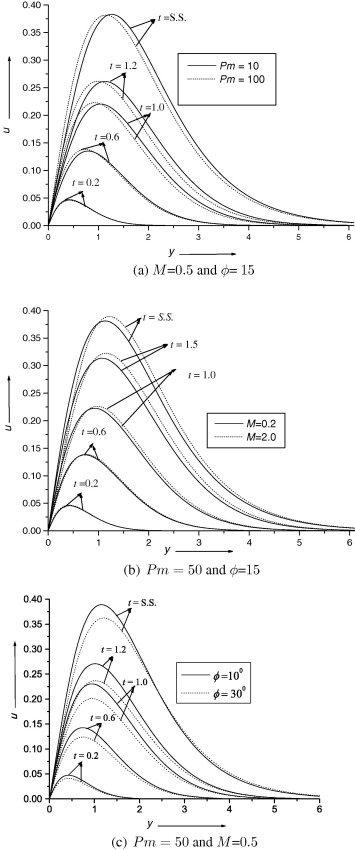Figure 4. Velocity profile at x = 1 for different values of ${\textstyle Pm{\mbox{,}}M}$ and ${\textstyle \phi }$ at Pr = 0.71.

Fig. 5 represents the variation in the x  -component of the induced magnetic field ${\textstyle H_{1}}$ with respect to y   for different values of ${\textstyle Pm{\mbox{,}}M{\mbox{,}}\phi }$ and t   at a fixed cross section ${\textstyle x=1.0}$. It is observed from Fig. 5(a) that the effect of Pm   on ${\textstyle H_{1}}$ is to reduce it. The reason behind this is that, increasing value of Pm is equivalent to decreasing magnetic diffusivity and consequently the strength of magnetic field becomes loose. Also, for higher value of Pm  , induced magnetic field (${\textstyle H_{1}}$) profiles are expanded widely while for low values of Pm these become shrink. The steady state is achieved much earlier for high values of Pm. The influence of M   on ${\textstyle H_{1}}$ component of induced magnetic field is shown in Fig. 5(b). Fig. 5(b) depicts that the influence of M   is to enhance the induced magnetic field ${\textstyle H_{1}}$ and reduce the magnetic boundary layer thickness. The steady state time reaches later with increase in M. Naturally, applied magnetic field perturbed the induced current, as a result induced magnetic field is produced in the fluid. Hence the resultant magnetic field enhances which can be noticed from Fig. 5(b). Fig. 5(c) presents the influence of semi-vertical angle (${\textstyle \phi }$) of the cone on induced magnetic field component ${\textstyle H_{1}}$ and the effect is found enhancing i.e. ${\textstyle H_{1}}$ component advances with increase in ${\textstyle \phi }$.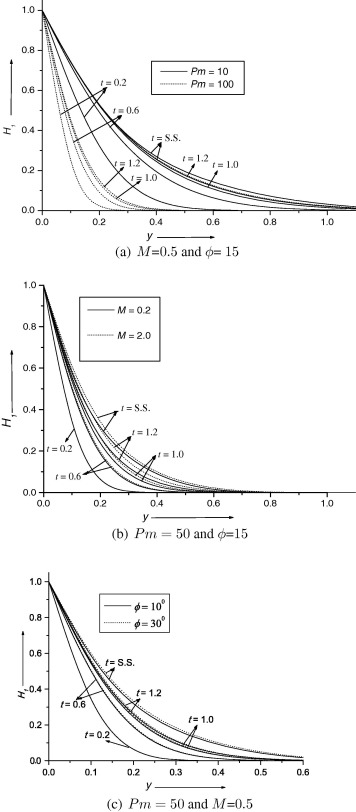Figure 5. Induced magnetic field profile ${\textstyle H_{1}}$ at x = 1 for different values of ${\textstyle Pm{\mbox{,}}M}$ and ${\textstyle \phi }$ at Pr = 0.71.

Fig. 6 shows the variation in the ${\textstyle y}$-component of the induced magnetic fields ${\textstyle \left(H_{2}\right)}$ with respect to y   for different values of ${\textstyle Pm{\mbox{,}}M{\mbox{,}}\phi }$ and t   at a fixed cross section ${\textstyle x=1.0}$. From Fig. 6(a), it can be clearly observed that the effect of Pm   on ${\textstyle H_{2}}$ is to reduce it, and as a consequence the magnetic boundary layer thickness increases. It can also be observed that for high value of ${\textstyle Pm{\mbox{,}}H_{2}}$ profiles increase monotonically and finally attain steady state but in case of low value of Pm, initially the profiles increase exponentially along y and after that monotonically and finally steady-state is achieved. Fig. 6(b) reveals that the normal component of induced magnetic field is more influenced by M. It decreases with increase in M. For high value of M, in beginning the profiles increase exponentially and after that there is gradual increase and finally steady state is achieved while for low values of M the profiles increase monotonically and become steady. In Fig. 6(c), we have shown the effect of semi-vertical angle ${\textstyle \phi }$ on y component of induced magnetic field. Fig. 6(c) displays that ${\textstyle H_{2}}$ profiles decrease with increase in ${\textstyle \phi }$. Also, induced magnetic field has increasing tendency with time. From the comparative study of Fig. 6(a) and (b), we noticed that time parameter influences strongly in case of increasing M. From Fig. 6, we can see that the normal component of induced magnetic field is monotonically increasing function of y. Figure 7 and Figure 8 show the variation in velocity and induced magnetic field profiles respectively at a fixed cross section ${\textstyle x=1}$ for different values of considered parameters for ${\textstyle Pr=7.0}$. The results are quite similar as velocity profile Fig. 4 and induced magnetic field profile Fig. 5 respectively.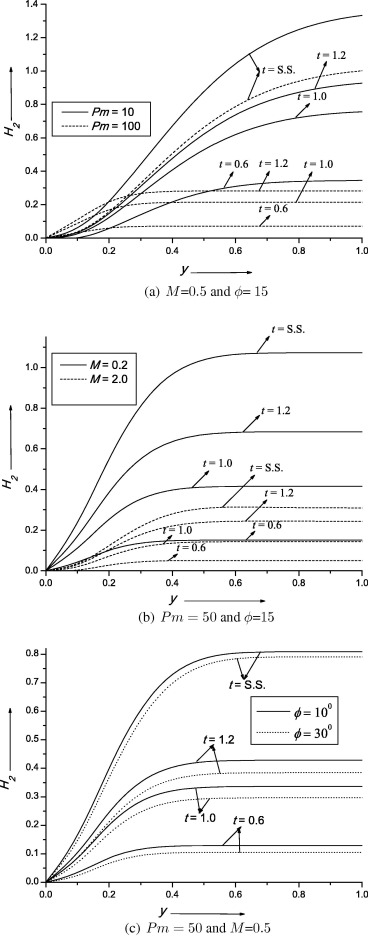Figure 6. Induced magnetic field profile ${\textstyle H_{2}}$ at x = 1 for different values of ${\textstyle Pm{\mbox{,}}M}$ and ${\textstyle \phi }$ at Pr = 0.71.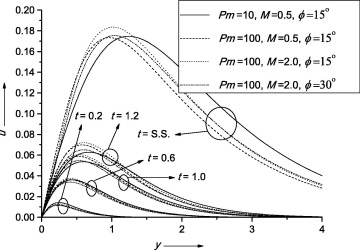Figure 7. Velocity profile at x = 1 for different values of ${\textstyle Pm{\mbox{,}}M}$ and ${\textstyle \phi }$ at Pr = 7.0.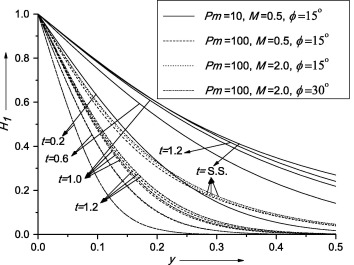Figure 8. Induced magnetic field profile at x = 1 for different values of ${\textstyle Pm{\mbox{,}}M}$ and ${\textstyle \phi }$ at Pr = 7.0.

Fig. 9(a) presents graphs for local skin-friction profiles. The effect of Pm is to reduce the local skin-friction profiles. For higher value of Pm profiles start increasing, achieve maximum value and then decrease gradually. But, in case of low Pm, there is a sharp decrease in skin-friction profiles. Also, the peak values shift toward the surface of the cone. In Fig. 9(b) we have shown the influence of magnetic parameter M and it is clear from Fig. 9(b) that M enhances the local skin-friction profiles. Also, the steady state is achieved later with the rise in M. The effect of semi-vertical angle of the cone on local skin-friction has been presented in Fig. 9(c). From Fig. 9(c), it can be seen that the effect of ${\textstyle \phi }$ is to reduce the local skin-friction profiles. From Fig. 9, we observed that the local skin-friction increases with time. Fig. 10 depicts for local Nusselt number and we can notice the qualitatively similar results as that for Fig. 9 but the effect of time parameter on it has opposite behavior i.e. the time parameter reduces the maximum value of local Nusselt number. From the comparative study of Figure 9 and Figure 10, we can say that the time parameter is more influential on the local skin-friction.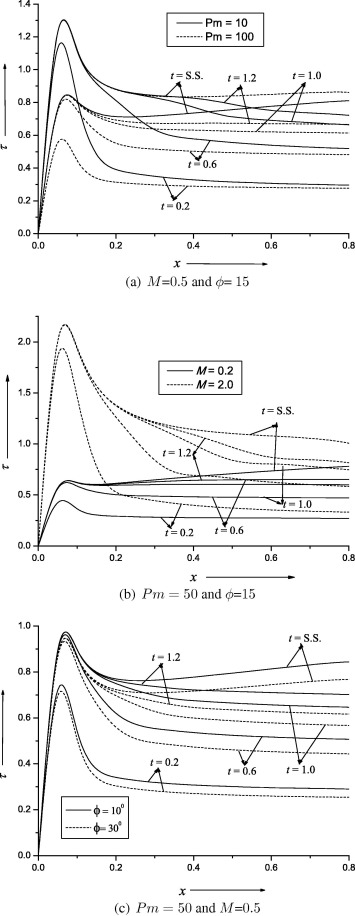Figure 9. Local skin-friction profile for different values of ${\textstyle Pm{\mbox{,}}M}$ and ${\textstyle \phi }$ at Pr = 0.71.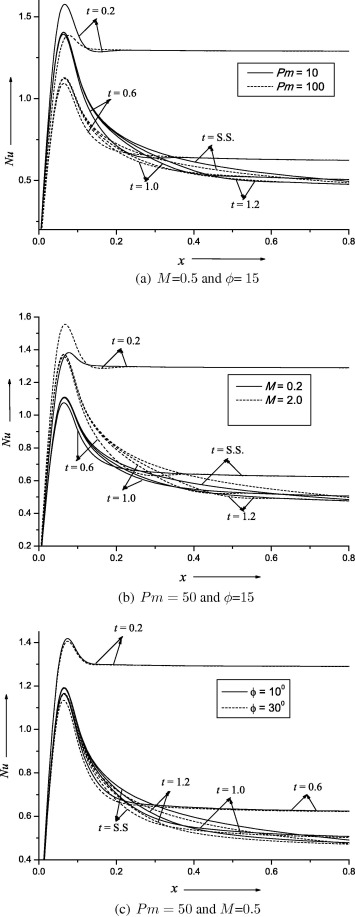Figure 10. Local Nusselt number profile for different values of ${\textstyle Pm{\mbox{,}}M}$ and ${\textstyle \phi }$ at Pr = 0.71.

Fig. 11 displays the average values of skin-friction for different value of ${\textstyle Pm{\mbox{,}}M{\mbox{,}}\phi }$ and t. Fig. 11(a) represents graph of ${\textstyle {\tau }_{av}}$ as a function of time. Here, we observed that there is retardation in average skin-friction profiles with increase in Pm but advancement with increase in M. Initially, these profiles start with minimum values and go on increasing with time, attain maximum and finally become steady. Fig. 11(b) shows sketches for average skin-friction profiles as a function of ${\textstyle \phi }$. It is revealed from the figure that average skin-friction profiles enhance with increase in magnetic parameter while magnetic Prandtl number has opposite effect. It can also be observed from the figure that the maximum value of ${\textstyle {\tau }_{av}}$ is achieved when ${\textstyle \phi }$ is 0 and it slightly decreases with increase in ${\textstyle \phi }$. Fig. 12(a) illustrates graph of average Nusselt number as a function of time. From Fig. 12(a), it can be seen that the average Nusselt number profiles get enlarged with rise in M but Pm put adverse effect. Fig. 12(b) depicts average Nusselt number as a function of ${\textstyle \phi }$. It is revealed from Fig. 12(b) that average Nusselt number profiles increase with increase in M but the rate of increase reduces when the values of Pm are simultaneously increased. Also, profiles decrease with increase in Pm  . Initially these are at maximum magnitude and slowly go down decreasing with increase in ${\textstyle \phi }$.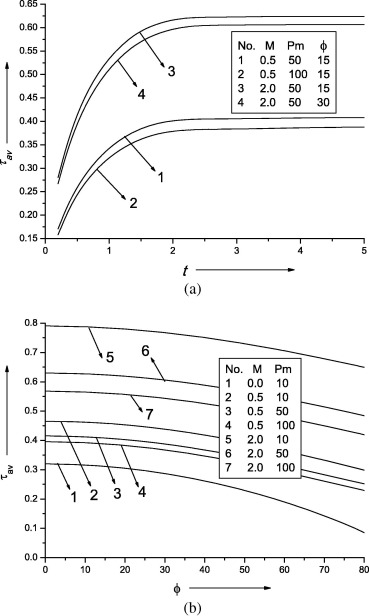Figure 11. Average skin-friction profile for different values of ${\textstyle Pm{\mbox{,}}M}$ and ${\textstyle \phi }$ at Pr = 0.71.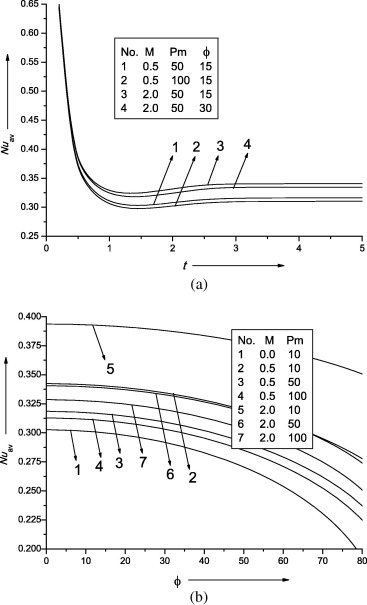Figure 12. Average Nusselt number for different values of ${\textstyle Pm{\mbox{,}}M}$ and ${\textstyle \phi }$ at Pr = 0.71.

In Table 2, we have shown the steady-state time for average skin-friction and average Nusselt number for different considered parameters. It is clearly visible from Table 2 that the steady-state time diminishes with enhancement in magnetic parameter while magnetic Prandtl number and the semi-vertical angle put adverse effect in achieving the steady-state time for both values of Pr = 0.71 and 7.0. The steady state time and average Nusselt number have increased with the value of Prandtl number but average skin-friction decreases.

Table 2. Steady State (S.S.) time for average skin-friction and average Nusselt number value for Pr = 0.71 and 7.0.
Pr M Pm ${\textstyle \phi }$ S.S. time ${\textstyle {\tau }_{av}}$ ${\textstyle {Nu}_{av}}$
0.71 0.0 15 3.7 0.311684 0.300058
0.71 0.2 50 15 4.6 0.347920 0.307195
0.71 2.0 50 15 4.1 0.599315 0.337142
0.71 0.5 10 15 3.8 0.441584 0.335434
0.71 0.5 100 15 5.8 0.379304 0.309285
0.71 0.5 50 0 4.4 0.403513 0.316820
0.71 0.5 50 30 4.7 0.373110 0.307153
7.0 0.5 10 15 6.7 0.340425 0.815505
7.0 0.5 100 15 9.7 0.273558 0.692389
7.0 2.0 100 15 9.3 0.449486 0.766056
7.0 2.0 100 30 10.6 0.437648 0.786713

Fig. 13 presents graphs for isotherms for different values of time and magnetic parameter M by keeping other physical parameters (Pm = 50 and ${\textstyle \phi }$ = 15) constant. From Fig. 13(a) and (b), we observe that for small values of time the isotherms are almost parallel indicating the conduction mode. But as the time is increased, the isotherms expanded which shows the convection process. Also, from the comparative study of Fig. 13(a)–(b), (c)–(d) and (e)–(f), we can see that the expansion in thermal boundary layers becomes reduced as the strength of magnetic field is increased. Fig. 14 presents graphs for isotherms for different values of time and Prandtl number Pr by keeping all other parameters constant. From Fig. 14(a), (c) and (e) it is clearly visible that as time increases isotherms get expanded which indicate that convection process increases with time. But from the comparative study of Fig. 14(a)–(b), (c)–(d) and (e)–(f), we observe that as value of Prandtl number rises up the thermal boundary layers become shrink which shows that convection is converting into conduction earlier. Fig. 15 depicts graphs for magnetic lines for different values of time and magnetic parameter M by keeping Pm   and ${\textstyle \phi }$ constant. For low values of time and M, the results are qualitatively similar to those of isotherms. But as the time increases, the effect of M is more pronounced in case of magnetic field lines. With the increase in value of M, the magnetic field lines become more expanded with lower values of contours which is a clear indication that the Lorentz force is more effective in this case.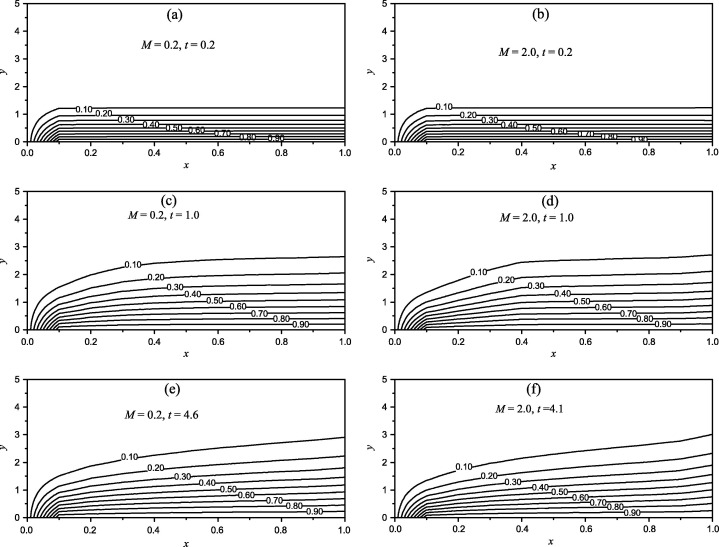Figure 13. Isotherms for different values of time and magnetic parameter for Pm = 50 and ${\textstyle \phi }$ = 15 at Ρr=0.71.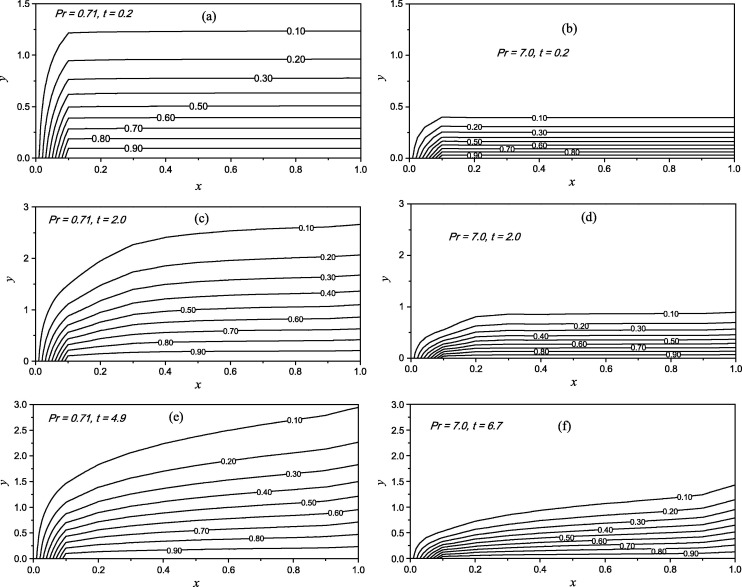Figure 14. Isotherms for different values of time and Prandtl number for Pm = 50, ${\textstyle M}$ = ${\textstyle 0.5}$ and ${\textstyle \phi }$ = 15.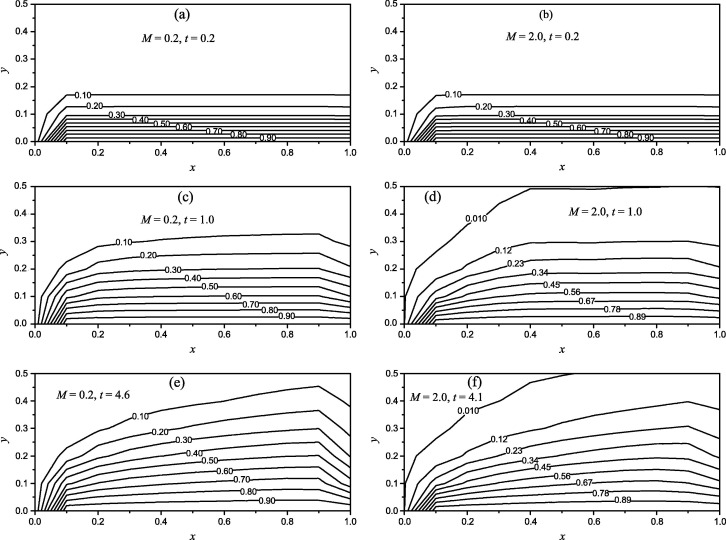Figure 15. Magnetic lines for different values of time and magnetic parameter at Pm = 50 and ${\textstyle \phi }$ = 15 at Pr = 0.71.

## 5. Conclusions

The transient free convective flow over a vertical cone under the influence of induced magnetic field has been studied. The dimensionless governing equations have been solved by using an implicit finite-difference method of Crank–Nicolson type. The present numerical results are compared with available results in the literature and are found to be in good agreement. By our study, we concluded the following results:

• With increase in value of Pm the momentum boundary layer thickness decreases while effect of M   and ${\textstyle \phi }$ is exactly opposite.
• The effect of Pm   on ${\textstyle H_{1}}$ is to reduce it as a consequence and magnetic boundary layer thickness decreases while effect of M   and ${\textstyle \phi }$ is to enhance it accordingly magnetic boundary layer thickness increases.
• ${\textstyle H_{2}}$ profiles decrease with the increase in all parameters.
• ${\textstyle {\tau }_{av}}$ and ${\textstyle {Nu}_{av}}$ increase with increase in M and decrease with increase in Pm   and ${\textstyle \phi }$.
• The time parameter increases the local skin-friction but trend is reversed for local Nusselt number.
• With increase in magnetic parameter, isotherms slightly shrink indicating slow convection process.
• The magnetic lines become expanded with increase in magnetic parameter for higher value of time.
• With increase in Pr isotherms become shrink but opposite behavior is observed in case of time parameter.
• Steady-state time for average skin-friction and average Nusselt number reduces with rise in magnetic parameter while magnetic Prandtl number and the semi-vertical angle have opposite effect.

### Document informationPublished on 12/04/17

Licence: Other

### Document Score0

Views 17
Recommendations 0